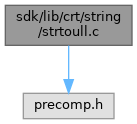ReactOS  0.4.15-dev-3177-gd6a0299
strtoull.c File Reference
`#include <precomp.h>`
Include dependency graph for strtoull.c:Go to the source code of this file.

## Functions

unsigned long long CDECL strtoull (const char *nptr, char **endptr, int base)

## ◆ strtoull()

 unsigned long long CDECL strtoull ( const char * nptr, char ** endptr, int base )

Definition at line 5 of file strtoull.c.

6 {
7  const char *s = nptr;
8  unsigned long long acc;
9  int c;
10  unsigned long long cutoff;
11  int neg = 0, any, cutlim;
12
13  /*
14  * See strtol for comments as to the logic used.
15  */
16  do {
17  c = *s++;
18  } while (isspace(c));
19  if (c == '-')
20  {
21  neg = 1;
22  c = *s++;
23  }
24  else if (c == '+')
25  c = *s++;
26  if ((base == 0 || base == 16) &&
27  c == '0' && (*s == 'x' || *s == 'X'))
28  {
29  c = s;
30  s += 2;
31  base = 16;
32  }
33  if (base == 0)
34  base = c == '0' ? 8 : 10;
35  cutoff = (unsigned long long)ULLONG_MAX / (unsigned long long)base;
36  cutlim = (unsigned long long)ULLONG_MAX % (unsigned long long)base;
37  for (acc = 0, any = 0;; c = *s++)
38  {
39  if (isdigit(c))
40  c -= '0';
41  else if (isalpha(c))
42  c -= isupper(c) ? 'A' - 10 : 'a' - 10;
43  else
44  break;
45  if (c >= base)
46  break;
47  if (any < 0 || acc > cutoff || (acc == cutoff && c > cutlim))
48  any = -1;
49  else {
50  any = 1;
51  acc *= base;
52  acc += c;
53  }
54  }
55  if (any < 0)
56  {
57  acc = ULLONG_MAX;
58 #ifndef _LIBCNT_
60 #endif
61  }
62  else if (neg)
63  acc = 0-acc;
64  if (endptr != 0)
65  *endptr = any ? (char *)((size_t)(s - 1)) : (char *)((size_t)nptr);
66  return acc;
67 }
errno_t __cdecl _set_errno(_In_ int _Value)
#define isspace(c)
Definition: acclib.h:69
#define isalpha(c)
Definition: acclib.h:74
GLuint base
Definition: 3dtext.c:35
unsigned char
Definition: typeof.h:29
#define isdigit(c)
Definition: acclib.h:68
__kernel_size_t size_t
Definition: linux.h:237
#define isupper(c)
Definition: acclib.h:71
const GLubyte * c
Definition: glext.h:8905
#define ERANGE
Definition: acclib.h:92
#define ULLONG_MAX
Definition: limits.h:47
GLdouble s
Definition: gl.h:2039
#define long
Definition: qsort.c:33
#define c
Definition: ke_i.h:80